gemseo / algos

# opt_problem module¶

Optimization problem.

The OptimizationProblem class operates on a DesignSpace defining:

• an initial guess $$x_0$$ for the design variables,

• the bounds $$l_b \leq x \leq u_b$$ of the design variables.

A (possible vector) objective function with a MDOFunction type is set using the objective attribute. If the optimization problem looks for the maximum of this objective function, the OptimizationProblem.change_objective_sign() changes the objective function sign because the optimization drivers seek to minimize this objective function.

Equality and inequality constraints are also MDOFunction instances provided to the OptimizationProblem by means of its OptimizationProblem.add_constraint() method.

The OptimizationProblem allows to evaluate the different functions for a given design parameters vector (see OptimizationProblem.evaluate_functions()). Note that this evaluation step relies on an automated scaling of function wrt the bounds so that optimizers and DOE algorithms work with inputs scaled between 0 and 1 for all the variables.

The OptimizationProblem has also a Database that stores the calls to all the functions so that no function is called twice with the same inputs. Concerning the derivatives’ computation, the OptimizationProblem automates the generation of the finite differences or complex step wrappers on functions, when the analytical gradient is not available.

Lastly, various getters and setters are available, as well as methods to export the Database to an HDF file or to a Dataset for future post-processing.

class gemseo.algos.opt_problem.OptimizationProblem(design_space, pb_type='non-linear', input_database=None, differentiation_method='user', fd_step=1e-07, parallel_differentiation=False, use_standardized_objective=True, **parallel_differentiation_options)[source]

Bases: object

An optimization problem.

Create an optimization problem from:

execute it from an algorithm provided by a DriverLib, and store some execution data in a Database.

In particular, this Database stores the calls to all the functions so that no function is called twice with the same inputs.

An OptimizationProblem also has an automated scaling of function with respect to the bounds of the design variables so that the driving algorithms work with inputs scaled between 0 and 1.

Lastly, OptimizationProblem automates the generation of finite differences or complex step wrappers on functions, when analytical gradient is not available.

Parameters:
• design_space (DesignSpace) – The design space on which the functions are evaluated.

• pb_type (str) –

The type of the optimization problem among OptimizationProblem.AVAILABLE_PB_TYPES.

By default it is set to “non-linear”.

• input_database (str | Path | Database | None) – A database to initialize that of the optimization problem. If None, the optimization problem starts from an empty database.

• differentiation_method (str) –

The default differentiation method to be applied to the functions of the optimization problem.

By default it is set to “user”.

• fd_step (float) –

The step to be used by the step-based differentiation methods.

By default it is set to 1e-07.

• parallel_differentiation (bool) –

Whether to approximate the derivatives in parallel.

By default it is set to False.

• use_standardized_objective (bool) –

Whether to use standardized objective for logging and post-processing.

By default it is set to True.

• **parallel_differentiation_options (int | bool) – The options to approximate the derivatives in parallel.

Add a callback function after each store operation or new iteration.

Parameters:
• callback_func (Callable) – A function to be called after some event.

• each_new_iter (bool) –

If True, then callback at every iteration.

By default it is set to True.

• each_store (bool) –

If True, then callback at every call to Database.store().

By default it is set to False.

Return type:

None

Add a constraint (equality and inequality) to the optimization problem.

Parameters:
• cstr_func (MDOFunction) – The constraint.

• value (float | None) – The value for which the constraint is active. If None, this value is 0.

• cstr_type (str | None) – The type of the constraint. Either equality or inequality.

• positive (bool) –

If True, then the inequality constraint is positive.

By default it is set to False.

Raises:
Return type:

None

Add an equality constraint to the optimization problem.

Parameters:
• cstr_func (MDOFunction) – The constraint.

• value (float | None) – The value for which the constraint is active. If None, this value is 0.

Return type:

None

Add an inequality constraint to the optimization problem.

Parameters:
• cstr_func (MDOFunction) – The constraint.

• value (float | None) – The value for which the constraint is active. If None, this value is 0.

• positive (bool) –

If True, then the inequality constraint is positive.

By default it is set to False.

Return type:

None

Add a function to be observed.

When the OptimizationProblem is executed, the observables are called following this sequence:

Parameters:
• obs_func (MDOFunction) – An observable to be observed.

• new_iter (bool) –

If True, then the observable will be called at each new iterate.

By default it is set to True.

Return type:

None

aggregate_constraint(constr_id, method='max', groups=None, **options)[source]

Aggregates a constraint to generate a reduced dimension constraint.

Parameters:
• constr_id (int) – The index of the constraint in constraints.

• method (str | Callable[[Callable], Callable]) –

The aggregation method, e.g. "max", "KS" or "IKS".

By default it is set to “max”.

• groups (tuple[ndarray] | None) – The groups for which to produce an output. If None, a single output constraint is produced.

• **options (Any) – The options of the aggregation method.

Raises:

ValueError – When the given is index is greater or equal than the number of constraints or when the method is aggregation unknown.

change_objective_sign()[source]

The OptimizationProblem expresses any optimization problem as a minimization problem. Then, an objective function originally expressed as a performance function to maximize must be converted into a cost function to minimize, by means of this method.

Return type:

None

check()[source]

Check if the optimization problem is ready for run.

Raises:

ValueError – If the objective function is missing.

Return type:

None

static check_format(input_function)[source]

Check that a function is an instance of MDOFunction.

Parameters:

input_function (Any) – The function to be tested.

Raises:

TypeError – If the function is not a MDOFunction.

Return type:

None

clear_listeners()[source]

Clear all the listeners.

Return type:

None

evaluate_functions(x_vect=None, eval_jac=False, eval_obj=True, eval_observables=False, normalize=True, no_db_no_norm=False, constraints_names=None, observables_names=None, jacobians_names=None)[source]

Compute the functions of interest, and possibly their derivatives.

These functions of interest are the constraints, and possibly the objective.

Some optimization libraries require the number of constraints as an input parameter which is unknown by the formulation or the scenario. Evaluation of initial point allows to get this mandatory information. This is also used for design of experiments to evaluate samples.

Parameters:
• x_vect (ndarray) – The input vector at which the functions must be evaluated; if None, the initial point x_0 is used.

• eval_jac (bool) –

Whether to compute the Jacobian matrices of the functions of interest. If True and jacobians_names is None then compute the Jacobian matrices (or gradients) of the functions that are selected for evaluation (with eval_obj, constraints_names, eval_observables andobservables_names). If False and jacobians_names is None then no Jacobian matrix is evaluated. If jacobians_names is not None then the value of eval_jac is ignored.

By default it is set to False.

• eval_obj (bool) –

Whether to consider the objective function as a function of interest.

By default it is set to True.

• eval_observables (bool) –

Whether to evaluate the observables. If True and observables_names is None then all the observables are evaluated. If False and observables_names is None then no observable is evaluated. If observables_names is not None then the value of eval_observables is ignored.

By default it is set to False.

• normalize (bool) –

Whether to consider the input vector x_vect normalized.

By default it is set to True.

• no_db_no_norm (bool) –

If True, then do not use the pre-processed functions, so we have no database, nor normalization.

By default it is set to False.

• constraints_names (Iterable[str] | None) – The names of the constraints to evaluate. If None then all the constraints are evaluated.

• observables_names (Iterable[str] | None) – The names of the observables to evaluate. If None and eval_observables is True then all the observables are evaluated. If None and eval_observables is False then no observable is evaluated.

• jacobians_names (Iterable[str] | None) – The names of the functions whose Jacobian matrices (or gradients) to compute. If None and eval_jac is True then compute the Jacobian matrices (or gradients) of the functions that are selected for evaluation (with eval_obj, constraints_names, eval_observables andobservables_names). If None and eval_jac is False then no Jacobian matrix is computed.

Returns:

The output values of the functions of interest, as well as their Jacobian matrices if eval_jac is True.

Raises:

ValueError – If a name in jacobians_names is not the name of a function of the problem.

Return type:

tuple[dict[str, float | ndarray], dict[str, ndarray]]

execute_observables_callback(last_x)[source]

The callback function to be passed to the database.

Call all the observables with the last design variables values as argument.

Parameters:

last_x (ndarray) – The design variables values from the last evaluation.

Return type:

None

export_hdf(file_path, append=False)[source]

Export the optimization problem to an HDF file.

Parameters:
• file_path (str | Path) – The path of the file to store the data.

• append (bool) –

If True, then the data are appended to the file if not empty.

By default it is set to False.

Return type:

None

export_to_dataset(name=None, by_group=True, categorize=True, opt_naming=True, export_gradients=False, input_values=None)[source]

Export the database of the optimization problem to a Dataset.

The variables can be classified into groups: Dataset.DESIGN_GROUP or Dataset.INPUT_GROUP for the design variables and Dataset.FUNCTION_GROUP or Dataset.OUTPUT_GROUP for the functions (objective, constraints and observables).

Parameters:
Returns:

A dataset built from the database of the optimization problem.

Return type:

Dataset

get_active_ineq_constraints(x_vect, tol=1e-06)[source]

For each constraint, indicate if its different components are active.

Parameters:
• x_vect (ndarray) – The vector of design variables.

• tol (float) –

The tolerance for deciding whether a constraint is active.

By default it is set to 1e-06.

Returns:

For each constraint, a boolean indicator of activation of its different components.

Return type:
get_all_functions()[source]

Retrieve all the functions of the optimization problem.

These functions are the constraints, the objective function and the observables.

Returns:

All the functions of the optimization problem.

Return type:
get_all_functions_names()[source]

Retrieve the names of all the function of the optimization problem.

These functions are the constraints, the objective function and the observables.

Returns:

The names of all the functions of the optimization problem.

Return type:

list[str]

get_best_infeasible_point()[source]

Retrieve the best infeasible point within a given tolerance.

Returns:

The best infeasible point expressed as the design variables values, the objective function value, the feasibility of the point and the functions values.

Return type:
get_constraints_names()[source]

Retrieve the names of the constraints.

Returns:

The names of the constraints.

Return type:

list[str]

get_constraints_number()[source]

Retrieve the number of constraints.

Returns:

The number of constraints.

Return type:

int

get_data_by_names(names, as_dict=True, filter_non_feasible=False)[source]

Return the data for specific names of variables.

Parameters:
• names (str | Iterable[str]) – The names of the variables.

• as_dict (bool) –

If True, return values as dictionary.

By default it is set to True.

• filter_non_feasible (bool) –

If True, remove the non-feasible points from the data.

By default it is set to False.

Returns:

The data related to the variables.

Return type:

ndarray | dict[str, ndarray]

get_design_variable_names()[source]

Retrieve the names of the design variables.

Returns:

The names of the design variables.

Return type:

list[str]

get_dimension()[source]

Retrieve the total number of design variables.

Returns:

The dimension of the design space.

Return type:

int

get_eq_constraints()[source]

Retrieve all the equality constraints.

Returns:

The equality constraints.

Return type:
get_eq_constraints_number()[source]

Retrieve the number of equality constraints.

Returns:

The number of equality constraints.

Return type:

int

get_eq_cstr_total_dim()[source]

Retrieve the total dimension of the equality constraints.

This dimension is the sum of all the outputs dimensions of all the equality constraints.

Returns:

The total dimension of the equality constraints.

Return type:

int

get_feasible_points()[source]

Retrieve the feasible points within a given tolerance.

This tolerance is defined by OptimizationProblem.eq_tolerance for equality constraints and OptimizationProblem.ineq_tolerance for inequality ones.

Returns:

The values of the design variables and objective function for the feasible points.

Return type:

tuple[list[ndarray], list[dict[str, float | list[int]]]]

get_function_dimension(name)[source]

Return the dimension of a function of the problem (e.g. a constraint).

Parameters:

name (str) – The name of the function.

Returns:

The dimension of the function.

Raises:
• ValueError – If the function name is unknown to the problem.

• RuntimeError – If the function dimension is not unavailable.

Return type:

int

get_function_names(names)[source]

Return the names of the functions stored in the database.

Parameters:

names (Iterable[str]) – The names of the outputs or constraints specified by the user.

Returns:

The names of the constraints stored in the database.

Return type:

list[str]

get_functions_dimensions(names=None)[source]

Return the dimensions of the outputs of the problem functions.

Parameters:

names (Iterable[str] | None) – The names of the functions. If None, then the objective and all the constraints are considered.

Returns:

The dimensions of the outputs of the problem functions. The dictionary keys are the functions names and the values are the functions dimensions.

Return type:

dict[str, int]

get_ineq_constraints()[source]

Retrieve all the inequality constraints.

Returns:

The inequality constraints.

Return type:
get_ineq_constraints_number()[source]

Retrieve the number of inequality constraints.

Returns:

The number of inequality constraints.

Return type:

int

get_ineq_cstr_total_dim()[source]

Retrieve the total dimension of the inequality constraints.

This dimension is the sum of all the outputs dimensions of all the inequality constraints.

Returns:

The total dimension of the inequality constraints.

Return type:

int

get_nonproc_constraints()[source]

Retrieve the non-processed constraints.

Returns:

The non-processed constraints.

Return type:
get_nonproc_objective()[source]

Retrieve the non-processed objective function.

Return type:

MDOFunction

get_number_of_unsatisfied_constraints(design_variables)[source]

Return the number of scalar constraints not satisfied by design variables.

Parameters:

design_variables (ndarray) – The design variables.

Returns:

The number of unsatisfied scalar constraints.

Return type:

int

get_objective_name(standardize=True)[source]

Retrieve the name of the objective function.

Parameters:

standardize (bool) –

Whether to use the name of the objective expressed as a cost, e.g. "-f" when the user seeks to maximize "f".

By default it is set to True.

Returns:

The name of the objective function.

Return type:

str

get_observable(name)[source]

Return an observable of the problem.

Parameters:

name (str) – The name of the observable.

Returns:

The pre-processed observable if the functions of the problem have already been pre-processed, otherwise the original one.

Return type:

MDOFunction

get_optimum()[source]

Return the optimum solution within a given feasibility tolerances.

Returns:

The optimum result, defined by:

• the value of the objective function,

• the value of the design variables,

• the indicator of feasibility of the optimal solution,

• the value of the constraints,

• the value of the gradients of the constraints.

Return type:
get_scalar_constraints_names()[source]

Return the names of the scalar constraints.

Returns:

The names of the scalar constraints.

Return type:

list[str]

get_violation_criteria(x_vect)[source]

Compute a violation measure associated to an iteration.

For each constraint, when it is violated, add the absolute distance to zero, in L2 norm.

If 0, all constraints are satisfied

Parameters:

x_vect (ndarray) – The vector of the design variables values.

Returns:

The feasibility of the point and the violation measure.

Return type:
get_x0_normalized(cast_to_real=False)[source]

Return the current values of the design variables after normalization.

Parameters:

cast_to_real (bool) –

Whether to cast the return value to real.

By default it is set to False.

Returns:

The current values of the design variables normalized between 0 and 1 from their lower and upper bounds.

Return type:

ndarray

has_constraints()[source]

Check if the problem has equality or inequality constraints.

Returns:

True if the problem has equality or inequality constraints.

has_eq_constraints()[source]

Check if the problem has equality constraints.

Returns:

True if the problem has equality constraints.

Return type:

bool

has_ineq_constraints()[source]

Check if the problem has inequality constraints.

Returns:

True if the problem has inequality constraints.

Return type:

bool

has_nonlinear_constraints()[source]

Check if the problem has non-linear constraints.

Returns:

True if the problem has equality or inequality constraints.

Return type:

bool

classmethod import_hdf(file_path, x_tolerance=0.0)[source]

Import an optimization history from an HDF file.

Parameters:
• file_path (str | Path) – The file containing the optimization history.

• x_tolerance (float) –

The tolerance on the design variables when reading the file.

By default it is set to 0.0.

Returns:

Return type:

OptimizationProblem

is_max_iter_reached()[source]

Check if the maximum amount of iterations has been reached.

Returns:

Whether the maximum amount of iterations has been reached.

Return type:

bool

is_point_feasible(out_val, constraints=None)[source]

Check if a point is feasible.

Note

If the value of a constraint is absent from this point, then this constraint will be considered satisfied.

Parameters:
• out_val (dict[str, ndarray]) – The values of the objective function, and eventually constraints.

• constraints (Iterable[MDOFunction] | None) – The constraints whose values are to be tested. If None, then take all constraints of the problem.

Returns:

The feasibility of the point.

Return type:

bool

preprocess_functions(is_function_input_normalized=True, use_database=True, round_ints=True, eval_obs_jac=False)[source]

Pre-process all the functions and eventually the gradient.

Required to wrap the objective function and constraints with the database and eventually the gradients by complex step or finite differences.

Parameters:
• is_function_input_normalized (bool) –

Whether to consider the function input as normalized and unnormalize it before the evaluation takes place.

By default it is set to True.

• use_database (bool) –

Whether to wrap the functions in the database.

By default it is set to True.

• round_ints (bool) –

Whether to round the integer variables.

By default it is set to True.

• eval_obs_jac (bool) –

Whether to evaluate the Jacobian of the observables.

By default it is set to False.

Return type:

None

static repr_constraint(func, ctype, value=None, positive=False)[source]

Express a constraint as a string expression.

Parameters:
• func (MDOFunction) – The constraint function.

• ctype (str) – The type of the constraint. Either equality or inequality.

• value (float | None) – The value for which the constraint is active. If None, this value is 0.

• positive (bool) –

If True, then the inequality constraint is positive.

By default it is set to False.

Returns:

A string representation of the constraint.

Return type:

str

reset(database=True, current_iter=True, design_space=True, function_calls=True, preprocessing=True)[source]

Partially or fully reset the optimization problem.

Parameters:
• database (bool) –

Whether to clear the database.

By default it is set to True.

• current_iter (bool) –

Whether to reset the current iteration OptimizationProblem.current_iter.

By default it is set to True.

• design_space (bool) –

Whether to reset the current point of the OptimizationProblem.design_space to its initial value (possibly none).

By default it is set to True.

• function_calls (bool) –

Whether to reset the number of calls of the functions.

By default it is set to True.

• preprocessing (bool) –

Whether to turn the pre-processing of functions to False.

By default it is set to True.

Return type:

None

AVAILABLE_PB_TYPES: ClassVar[str] = ['linear', 'non-linear']
COMPLEX_STEP: Final[str] = 'complex_step'
CONSTRAINTS_GROUP: Final[str] = 'constraints'
DESIGN_SPACE_ATTRS: Final[str] = ['u_bounds', 'l_bounds', 'x_0', 'x_names', 'dimension']
DESIGN_SPACE_GROUP: Final[str] = 'design_space'
DESIGN_VAR_NAMES: Final[str] = 'x_names'
DESIGN_VAR_SIZE: Final[str] = 'x_size'
DIFFERENTIATION_METHODS: ClassVar[str] = ['user', 'complex_step', 'finite_differences', 'no_derivatives']
FINITE_DIFFERENCES: Final[str] = 'finite_differences'
FUNCTIONS_ATTRS: ClassVar[str] = ['objective', 'constraints']
GGOBI_FORMAT: Final[str] = 'ggobi'
HDF5_FORMAT: Final[str] = 'hdf5'
KKT_RESIDUAL_NORM: Final[str] = 'KKT residual norm'
LINEAR_PB: Final[str] = 'linear'
NON_LINEAR_PB: Final[str] = 'non-linear'
NO_DERIVATIVES: Final[str] = 'no_derivatives'
OBJECTIVE_GROUP: Final[str] = 'objective'
OBSERVABLES_GROUP: Final[str] = 'observables'
OPTIM_DESCRIPTION: ClassVar[str] = ['minimize_objective', 'fd_step', 'differentiation_method', 'pb_type', 'ineq_tolerance', 'eq_tolerance']
OPT_DESCR_GROUP: Final[str] = 'opt_description'
SOLUTION_GROUP: Final[str] = 'solution'
activate_bound_check: ClassVar[bool] = True

Whether to check if a point is in the design space before calling functions.

constraint_names: dict[str, list[str]]

The standardized constraint names bound to the original ones.

constraints: list[gemseo.core.mdofunctions.mdo_function.MDOFunction]

The constraints.

database: Database

The database to store the optimization problem data.

design_space: DesignSpace

The design space on which the optimization problem is solved.

property differentiation_method: str

The differentiation method.

property dimension: int

The dimension of the design space.

eq_tolerance: float

The tolerance for the equality constraints.

fd_step: float

The finite differences step.

ineq_tolerance: float

The tolerance for the inequality constraints.

property is_mono_objective: bool

Whether the optimization problem is mono-objective.

minimize_objective: bool

Whether to maximize the objective.

new_iter_observables: list[gemseo.core.mdofunctions.mdo_function.MDOFunction]

The observables to be called at each new iterate.

nonproc_constraints: list[gemseo.core.mdofunctions.mdo_function.MDOFunction]

The non-processed constraints.

nonproc_new_iter_observables: list[gemseo.core.mdofunctions.mdo_function.MDOFunction]

The non-processed observables to be called at each new iterate.

nonproc_objective: MDOFunction

The non-processed objective function.

nonproc_observables: list[gemseo.core.mdofunctions.mdo_function.MDOFunction]

The non-processed observables.

property objective: MDOFunction

The objective function.

observables: list[gemseo.core.mdofunctions.mdo_function.MDOFunction]

The observables.

property parallel_differentiation: bool

Whether to approximate the derivatives in parallel.

property parallel_differentiation_options: bool

The options to approximate the derivatives in parallel.

pb_type: str

The type of optimization problem.

preprocess_options: dict

The options to pre-process the functions.

solution: OptimizationResult

The solution of the optimization problem.

stop_if_nan: bool

Whether the optimization stops when a function returns NaN.

use_standardized_objective: bool

Whether to use standardized objective for logging and post-processing.

The standardized objective corresponds to the original one expressed as a cost function to minimize. A DriverLib works with this standardized objective and the Database stores its values. However, for convenience, it may be more relevant to log the expression and the values of the original objective.

## Examples using OptimizationProblem¶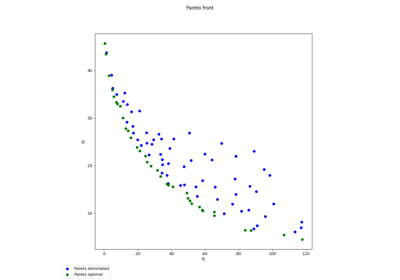Pareto front on Binh and Korn problem

Pareto front on Binh and Korn problem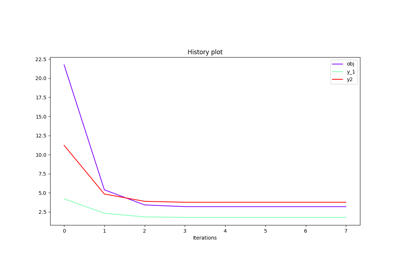Store observables

Store observables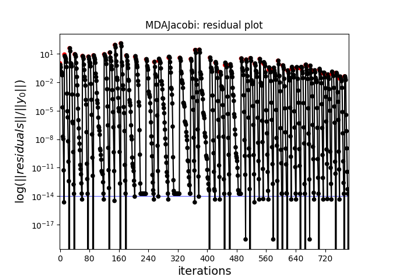BiLevel-based MDO on the Sobieski SSBJ test case

BiLevel-based MDO on the Sobieski SSBJ test caseDataset from an optimization problem

Dataset from an optimization problem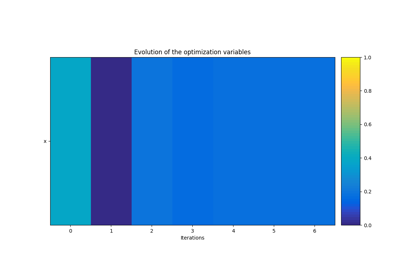Analytical test case # 2

Analytical test case # 2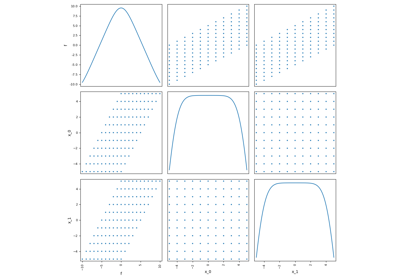Analytical test case # 3

Analytical test case # 3Use a design of experiments from a file

Use a design of experiments from a fileUse a design of experiments from an array

Use a design of experiments from an array